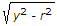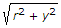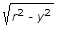# Civil Engineering - Applied Mechanics

### Exercise :: Applied Mechanics - Section 4

11.

One half of a vibration of a body, is called

 A. period time B. oscillation C. beat D. amplitude.

Explanation:

No answer description available for this question. Let us discuss.

12.

On a ladder resisting on a smooth ground and leaning against a rough vertical wall, the force of friction acts

 A. towards the wall at its upper end B. away from the wall at its upper end C. upwards at its upper end D. downwards at its upper end E. none of these.

Explanation:

No answer description available for this question. Let us discuss.

13.

The acceleration of a particle moving along the circumference of a circle with a uniform speed, is directed

 A. radially B. tangentially at that point C. away from the centre D. towards the centre.

Explanation:

No answer description available for this question. Let us discuss.

14.

From the circular plate of a diameter 6 cm is cut out a circular plate whose diameter is equal to radius of the plate. The c.g. of the remainder shifts from the original position through

 A. 0.25 cm B. 0.50 cm C. 0.75 cm D. 1.00 cm.

Explanation:

No answer description available for this question. Let us discuss.

15.

If a particle moves with a uniform angular velocity ω radians/sec along the circumference of a circle of radius r, the equation for the velocity of the particle, is

 A. v = ωB. y = ω y - r C. v = ωD. v = ω